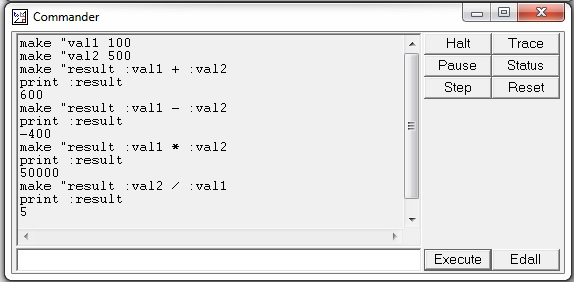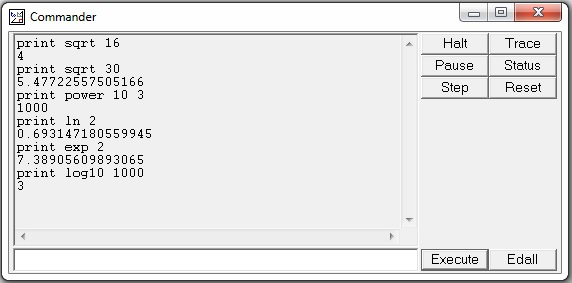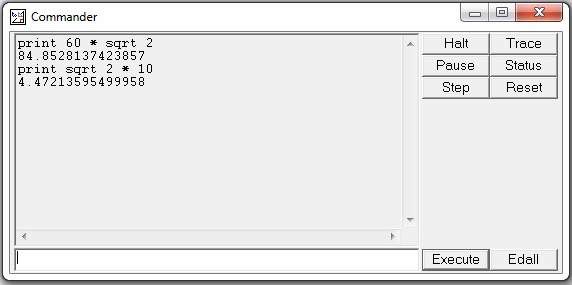# Logo - Arithmetic Operations

#### Simulation with LOGO!Soft

48 Lectures 6 hours

#### Become A Logo Designer

38 Lectures 2.5 hours

#### Professional Logo Design

81 Lectures 7.5 hours

Logo provides the usual arithmetic operations of addition, subtraction, multiplication and division, denoted by the symbols +, -, *, /. Each of these operations produces a result. If you don't do something with the result, such as print it, Logo will show an error.

With the print command, the result of an arithmetic operation can be used and printed in the command window. Examples given in the following screenshot demonstrate the same.Other useful commands are −

• sqrt − It takes one non-negative argument and returns its square root.

• power − It takes two arguments, call them ‘a’ and ‘b’, and generates a to the b power.

• ln − It takes one argument and returns its natural logarithm.

• exp − It takes one argument and computes e to that power, e is the natural number 2.718281828.

• log10 − It takes the logarithm to base 10 of its one argument.

Following screenshot shows an example of the above commands with their respective output.Arithmetic operators have a precedence that determines the order with which they are evaluated.

Note − print 60 * sqrt 2 and print sqrt 2 * 60 produce different answers. Here the * operator has a precedence over the sqrt operator. Thus, * will be done before sqrt, if there is a choice, as there is in the second case.

For this reason, the first statement prints the value of 60 times the square root of 2, whereas the second statement prints the square root of 120 as shown in the following screenshot.